## Friday, 8 August 2014

### CHAPTER 17-Properties of Dot Product

From the definition of the dot product, we can make certain useful observations about its properties.
(i) The angle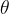$\theta$ between two vectors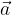$\vec a$ and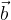$\vec b$ is given by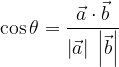$\cos \theta = \dfrac{{\vec a \cdot \vec b}}{{\left| {\vec a} \right|\;\left| {\vec b} \right|}}$
(ii)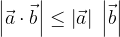$\left| {\vec a \cdot \vec b} \right| \le \left| {\vec a} \right|\;\left| {\vec b} \right|$, the equality holding only if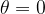$\theta = 0$ or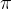$\pi$
(iii) The projection of$\vec a$ on$\vec b$ is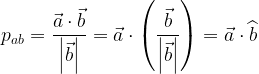${p_{ab}} = \dfrac{{\vec a \cdot \vec b}}{{\left| {\vec b} \right|}} = \vec a \cdot \left( {\dfrac{{\vec b}}{{\left| {\vec b} \right|}}} \right) = \vec a \cdot \widehat b$
(iv) The projection of$\vec b$ on$\vec a$ is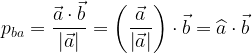${p_{ba}} = \dfrac{{\vec a \cdot \vec b}}{{\left| {\vec a} \right|}} = \left( {\dfrac{{\vec a}}{{\left| {\vec a} \right|}}} \right) \cdot \vec b = \widehat a \cdot \vec b$
(v) Scalar product is commutative i.e.,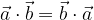$\vec a \cdot \vec b = \vec b \cdot \vec a$
(vi) Scalar product is distributive i.e.,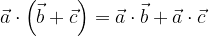$\vec a \cdot \left( {\vec b + \vec c} \right) = \vec a \cdot \vec b + \vec a \cdot \vec c$ and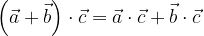$\left( {\vec a + \vec b} \right) \cdot \vec c = \vec a \cdot \vec c + \vec b \cdot \vec c$
(vi) The scalar product of two vectors is zero if and only if the two vectors are perpendicular.
This also gives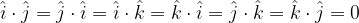$\hat i \cdot \hat j = \hat j \cdot \hat i = \hat i \cdot \hat k = \hat k \cdot \hat i = \hat j \cdot \hat k = \hat k \cdot \hat j = 0$
(vii) For any vector$\vec a$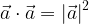${\vec a \cdot \vec a = {{\left| {\vec a} \right|}^2}}$
Thus,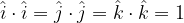$\hat i \cdot \hat i = \hat j \cdot \hat j = \hat k \cdot \hat k = 1$
(viii)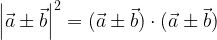${\left| {\vec a \pm \vec b} \right|^2} = (\vec a \pm \vec b) \cdot (\vec a \pm \vec b)$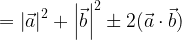$= {\left| {\vec a} \right|^2} + {\left| {\vec b} \right|^2} \pm 2(\vec a \cdot \vec b)$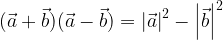$(\vec a + \vec b)(\vec a - \vec b) = {\left| {\vec a} \right|^2} - {\left| {\vec b} \right|^2}$
(ix) This property is very important. If two vectors$\vec a$ and$\vec b$ have been specified in rectangular form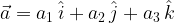$\vec a = {a_1}\,\hat i + {a_2}\,\hat j + {a_3}\,\hat k$ and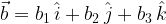$\vec b = {b_1}\,\hat i + {b_2}\,\hat j + {b_3}\,\hat k$ then
We have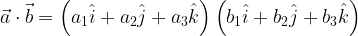$\vec a \cdot \vec b = \left( {{a_1}\hat i + {a_2}\hat j + {a_3}\hat k} \right)\left( {{b_1}\hat i + {b_2}\hat j + {b_3}\hat k} \right)$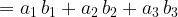$= {a_1}\,{b_1} + {a_2}\,{b_2} + {a_3}\,{b_3}$ Using properties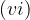$(vi)$ and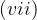$(vii)$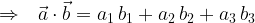$\Rightarrow\,\,\,\,{\vec a \cdot \vec b = {a_1}\,{b_1} + {a_2}\,{b_2} + {a_3}\,{b_3}}$
The angle$\theta$ between the two vectors will be given by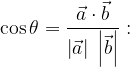$\cos \theta = \dfrac{{\vec a \cdot \vec b}}{{\left| {\vec a} \right|\;\left| {\vec b} \right|}}:$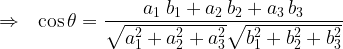$\Rightarrow \,\,\,\, \cos \theta = \dfrac{{{a_1}{\kern 1pt} \,{b_1} + {a_2}\,{b_2} + {a_3}\,{b_3}}}{{\sqrt {a_1^2 + a_2^2 + a_3^2} \sqrt {b_1^2 + b_2^2 + b_3^2} }}$
(x) The direction cosines$l$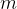$m$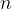$n$ of a vector$\vec a$ will be given by$l = \hat a \cdot \hat i,\;\;\;m = \hat a \cdot \hat j,\;\;\;\;n = \hat a \cdot \hat k$
(xi) Let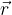$\vec r$ be a vector coplanar with the vectors$\vec a$ and$\vec b$. If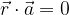$\vec r \cdot \vec a = 0$ and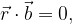$\vec r \cdot \vec b = 0,$ this would imply that$\vec r$ is perpendicular to both$\vec a$ and$\vec b$. This can only happen if$\vec a$ and$\vec b$ are collinear.
Analogously, let$\vec r$ be an arbitrary vector and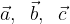$\vec a,\;\;\vec b,\;\;\vec c$ be three vectors such that$\vec r \cdot \vec a = \vec r \cdot \vec b = \vec r \cdot \vec c = 0$
This means that$\vec r$ is perpendicular to each of$\vec a$$\vec b$ and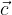$\vec c$ which can only happen if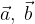$\vec a,\;\vec b$ and$\vec c$ are coplanar.
(xii) Let$\vec a,\;\;\vec b,\;\;\vec c$ be three non-coplanar vectors. We’ve already discussed that$\vec a,\;\;\vec b,\;\;\vec c$ can form a basis for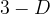$3 - D$ space. Any vector$\vec r$ can be written in this basis as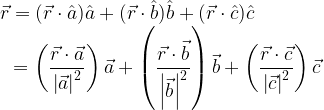$\begin{array}{l} \vec r = (\vec r \cdot \hat a)\hat a + (\vec r \cdot \hat b)\hat b + (\vec r \cdot \hat c)\hat c\\ \,\,\,\, = \left( {\dfrac{{\vec r \cdot \vec a}}{{{{\left| {\vec a} \right|}^2}}}} \right)\vec a + \left( {\dfrac{{\vec r \cdot \vec b}}{{{{\left| {\vec b} \right|}^2}}}} \right)\vec b + \left( {\dfrac{{\vec r \cdot \vec c}}{{{{\left| {\vec c} \right|}^2}}}} \right)\vec c \end{array}$
This representation is of significant importance and you must understand how it comes about.